# Psychrometric Processes

#### A view of psychrometric chart is shown:

Qc = ma (h2 − h1 ) = mapm (T2 − T1 )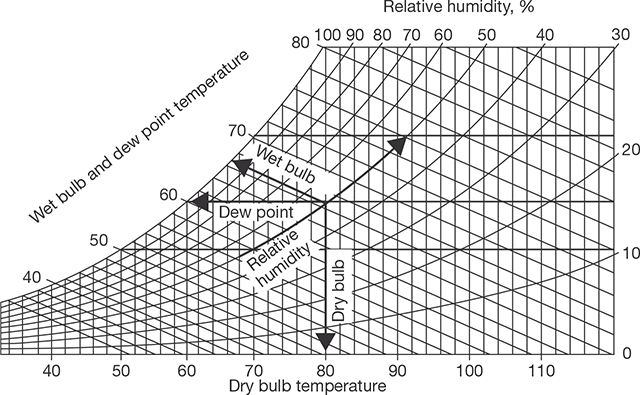Psychrometric Chart

#### The different psychrometric processes are shown: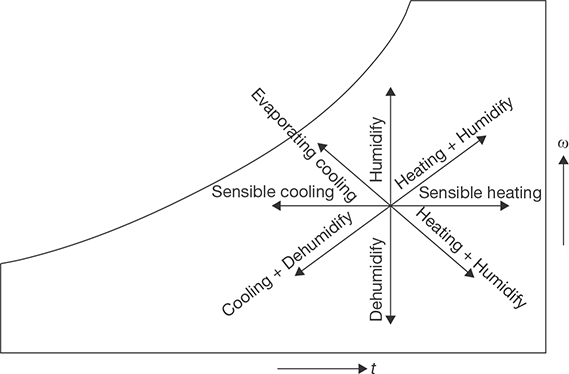Psychrometric Processes

#### Sensible Cooling

During this process, the moisture content of air remains constant but its temperature decreases as it passes over a cooling coil. To keep the moisture content constant, the surface of the cooling coil should be dry and its surface temperature should be greater than the dew point temperature of the air. If the cooling coil is 100% effective, then the exit temperature of the air will be equal to the coil temperature. However, in practice, the exit air temperature will be higher than the cooling coil temperature. Figure below shows the sensible cooling process 2–1 on a psychrometric chart. The heat rejection rate during this process is given by: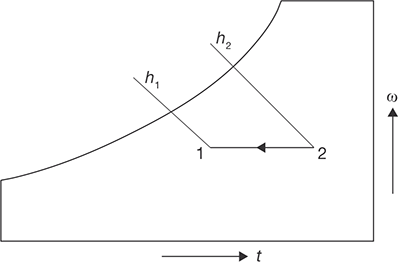Sensible Cooling Process 2-1 on Psychrometric Chart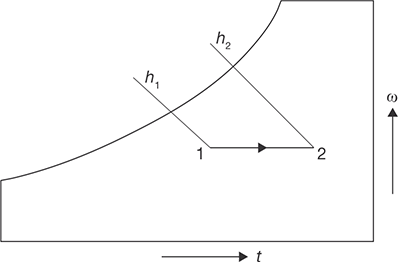Sensible Heating Process 1-2 on Psychrometric Chart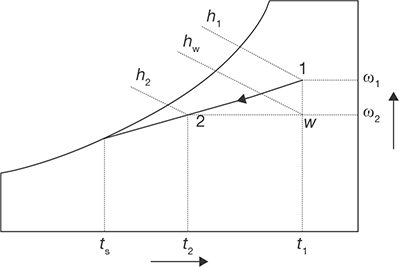Cooling and Dehumidification Process

#### Sensible Heating

During this process, the moisture content of air remains constant and its temperature increases as it flows over a heating coil. The heat addition rate during this process is given by:

Qh = ma (h2 − h1 ) = mapm (T2 − T1 )

where cpm is the humid specific heat (≈1.0216 kJ/kg dry air) and ma is the mass flow rate of dry air (kg/s).

#### Cooling and Dehumidification

When moist air is cooled below its dew-point by bringing it in contact with a cold surface, some of the water vapor in the air condenses and leaves the air stream as a liquid, as a result, both the temperature and humidity ratio of air decreases as shown. This is the process air undergoes in an air conditioning system. The actual process path depends on the type of cold surface, the surface temperature, and flow conditions, but for simplicity, the process line is assumed to be a straight line as shown in Figure 8.11. The heat and mass transfer rates can be expressed in terms of the initial and final conditions by applying the conservation of mass and conservation of energy equations as given below:

By applying mass balance for the water:

ma .ωa = ma .ω2 + mw

By applying energy balance:

ma.ha = Qr + mw.hw + ma.h2

From the above two equations, the load on the cooling coil, Qt is given by:

Qr = ma (h1 − h2 ) − ma ( ω1 − ω2 )hw

The 2nd term on the RHS of the above equation is normally small compared to the other terms, so it can be neglected. Hence,

Qr = ma (h1 − h2 )

It can be observed that the cooling and de-humidification process involves both latent and sensible heat transfer processes, hence, the total, latent and sensible heat transfer rates (QrQl, and Qs) can be written as:

Qr = Ql +Qs

where Ql = ma (h1 − hw ) = ma.hfg (ω1 − ωw )

and Qs = ma (hw − h2 ) = ma.cpm (T1 − T2 )

#### Sensible Heat Factor (SHF)

It is defined as the ratio of sensible to total heat transfer rate (Qt), i.e.,

SHF = Qs / Qt = Qs / (Qs + Ql )

From the above equation, we can observe that an SHF of 1.0 corresponds to no latent heat transfer and an SHF of 0 corresponds to no sensible heat transfer. An SHF of 0.75 to 0.80 is quite common in air conditioning systems in a normal dry-climate. A lower value of SHF, say 0.6, implies a high latent heat load such as that occurs in a humid climate.

The temperature, Ts, is the effective surface temperature of the cooling coil and is known as apparatus dew-point (ADP) temperature. In an ideal situation, when all the air comes in perfect contact with the cooling coil surface, then the exit temperature of the air will be same as ADP of the coil. However, in the actual case, the exit temperature of the air will always be greater than the apparatus dew-point temperature due to boundary layer development as air flows over the cooling coil surface and also due to temperature variation along the fins, etc. Hence, we can define a by-pass factor (BPF) as it can be easily seen that higher the by-pass factor larger will be the difference between air outlet temperature and the cooling coil temperature. When BPF is 1.0, all the air bypasses the coil and there will not be any cooling or de-humidification.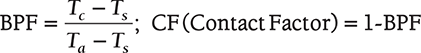Where Tc temperature of air leaving, Ta is temperature of air entering and Ts is temperature of surface of cooling coil.

#### Heating and Humidification

During winter it is essential to heat and humidity the room air for comfort. This is normally done by first sensible heating the air and then adding water vapor to the air stream through steam nozzles.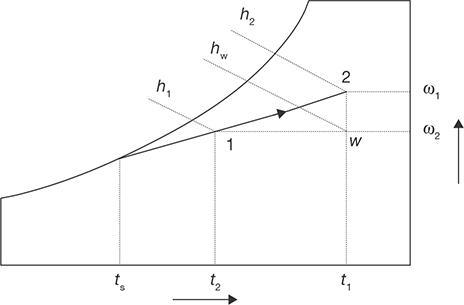Heating and Humidification Process

Mass balance of water vapor for the control volume yields the rate, at which steam has to be added, i.e., mw:

mw = ma (ω2 − ω1)

where ma is the mass flow rate of dry air. From energy balance:

Qh = ma (h2 − h1) − mwhw

where Qh is the heat supplied through the heating coil and hw is the enthalpy of steam. Since this process also involves simultaneous heat and mass transfer, we can define a sensible heat factor for the process in a way similar to that of a cooling and dehumidification process.

#### Cooling and Humidification

As the name implies, during this process, the air temperature drops and its humidity increases. This can be achieved by spraying cool water in the air stream. The temperature of the water should be lower than the dry-bulb temperature of air but higher than its dew-point temperature to avoid condensation (TDPT < T2 < T1).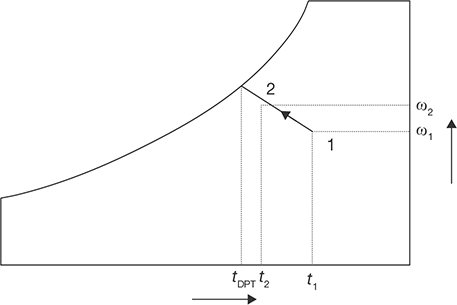Cooling and Humidification Process

During this process, there is sensible heat transfer from air to water and latent heat transfer from water to air. Hence, the total heat transfer depends upon the water temperature. If the temperature of the water sprayed is equal to the wet-bulb temperature of the air, then the net transfer rate will be zero as the sensible heat transfer from air to water will be equal to latent heat transfer from water to air. If the water temperature is greater than WBT, then there will be a net heat transfer from water to air. If the water temperature is less than WBT, then the net heat transfer will be from air to water. Under a special case when the spray water is entirely recirculated and is neither heated nor cooled, the system is perfectly insulated and the make-up water is supplied at WBT, then at steady-state, the air undergoes an adiabatic saturation process, during which its WBT remains constant. This is the process of adiabatic saturation. The process of cooling and humidification is encountered in a wide variety of devices such as evaporative coolers, cooling towers, etc.

#### Heating and Dehumidification

This process can be achieved by using a hygroscopic material, which absorbs or adsorbs the water vapor from the moisture. If this process is thermally isolated, then the enthalpy of air remains constant, as a result, the temperature of air increases as its moisture content decreases. This hygroscopic material can be a solid or a liquid. In general, the absorption of water by the hygroscopic material is an exothermic reaction, as a result heat is released during this process, which is transferred to air and the enthalpy of air increases.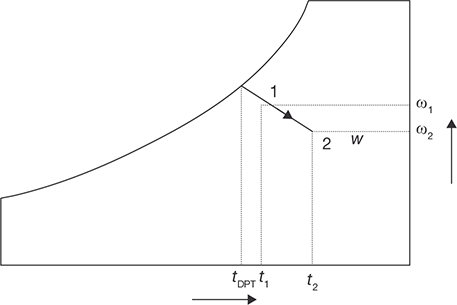Heating Dehumidification Process